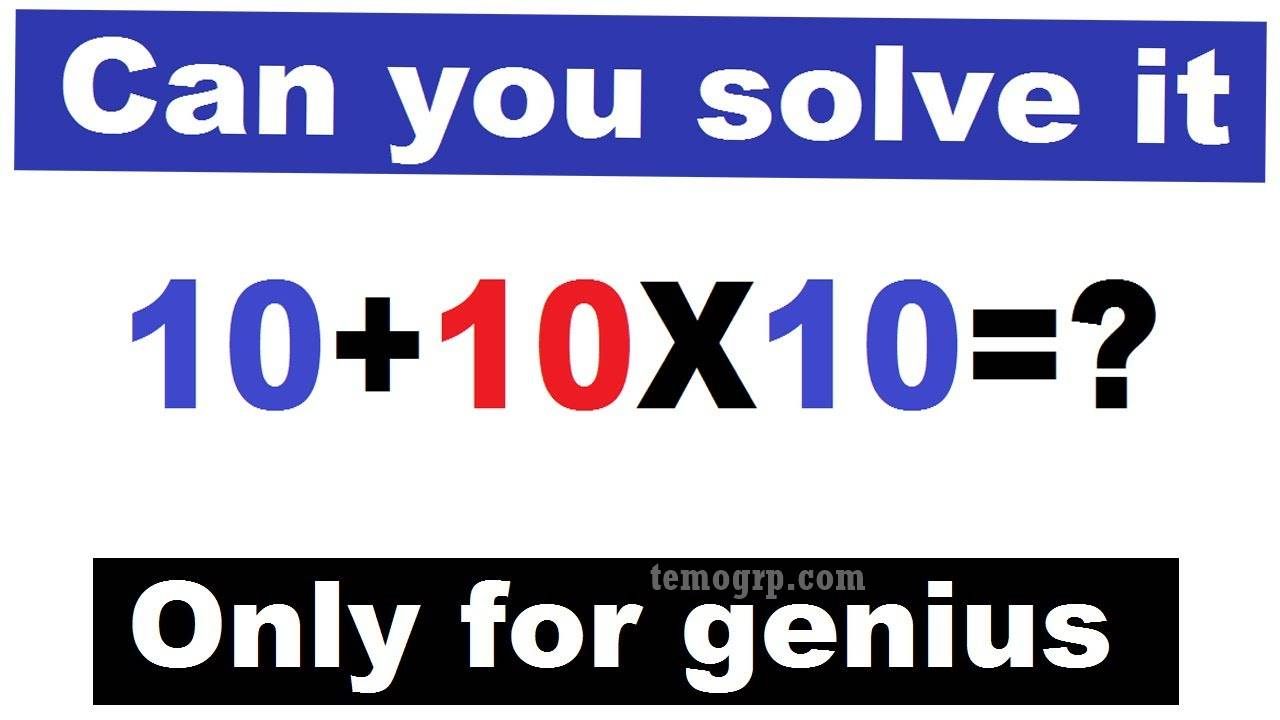How is 10-10x10+10 = -80? Answer or Solution Explained Step-By-Step! What is is 10-10x10+10? Solution to 10-10x10+10 = -80.

Home >> >> >> How is 10-10x10+10 = -80? Answer/Solution Explained!

The answer or solution to how is 10 -10 × 10 + 10 = -80 looks simple but could be tricky. Many folks always tend to ignore basic mathematical principles. PEMDAS and BODMAS are core Maths rules that must be used to solve this simple problem.

### What is the meaning of PEMDAS?

PEMDAS stands for:

• P= Parentheses
• E= Exponents
• M= Multiplication
• S= Subtraction.

### What is the meaning of BODMAS?

The whole meaning of BODMAS is:

• B=Bracket
• O=Order of operation
• D=Division
• M=Multiplication
• S=Subtraction

## How is 10-10x10+10 = -80? Answer or Solution Explained Step-By-Step!

We shall solve the question 10-10x10+10 = -80 using PEMDAS and BODMAS below.

### A. Solution to 10-10x10+10 = -80  Using the PEMDAS Method

• PEMDAS says you would do parentheses, exponents, multiplication/division, and addition/subtraction.
• There are no parentheses or exponents in the problem, but there is multiplication. Thus, you would do 10*10 first, so it would be 10–100+10.
• Now that everything is just addition/subtraction, you would do the problem from left to right.
• 10–100+10 = -90+10
• -90+10= -80.

### B. Solution to 10-10x10+10 = -80  Using the BODMAS Method

• According to the BODMAS rule, multiplication has to be performed first.
• So, the equation becomes 10–100+10.
• Next comes the addition of the numbers: 20–100
• And the last step will be subtraction: -80
• So, -80 is the correct answer.Name

ltr
item
Temo Group: How is 10-10x10+10 = -80? Answer/Solution Explained!
How is 10-10x10+10 = -80? Answer/Solution Explained!
How is 10-10x10+10 = -80? Answer or Solution Explained Step-By-Step! What is is 10-10x10+10? Solution to 10-10x10+10 = -80.